# Samacheer Kalvi 10th Science Solutions Chapter 11 Carbon and its Compounds

Get Updated Tamilnadu State Board Solutions for Class 10 Science Solutions Chapter 11 Carbon and its Compounds Questions and Answer in PDF Format and download them free of cost. Samacheer Kalvi Class 10 Science Solutions Chapter 11 Carbon and its Compounds provided are as per the latest exam pattern and syllabus. Access the topics of Chapter 11 Carbon and its Compounds through the direct links available depending on the need. Clear all your queries on the Class 10 Science Subject by using the Samacheer Kalvi Board Solutions for Chapter 11 Carbon and its Compounds existing.

## Tamilnadu Samacheer Kalvi 10th Science Solutions Chapter 11 Carbon and its Compounds

If you are eager to know about the Tamilnadu State Board Solutions of Class 10 Science Solutions Chapter 11 Carbon and its Compounds Questions and Answer you will find all of them here. You can identify the knowledge gap using these Tamilnadu State Board Class 10 Science Solutions PDF and plan accordingly. Don’t worry about the accuracy as they are given after extensive research by people having subject knowledge alongside from the latest Science Solutions Textbooks.

### Samacheer Kalvi 10th Science Carbon and its Compounds Textual Evaluation Solved

I. Choose the best answer.

Carbon And Its Compounds Class 10 Solutions Question 1.
The molecular formula of an open – chain organic compound is C3H6. The class of the compound is ______.
(a) alkane
(b) alkene
(c) alkyne
(d) alcohol.
Answer:
(b) alkene
Hint:
As we know the general molecular formula of alkene is CnH2n.
n = 2, C2H4
n = 3, C3H6
n = 4, C4H8

Class 10 Carbon And Its Compounds Solutions Question 2.
The IUPAC name of an organic compound is 3-Methyl butan-1-ol. What type of compound it is?
(a) Aldehyde
(b) Carboxylic acid
(c) Ketone
(d) Alcohol.
Answer:
(d) Alcohol
3-Methyl butan-1-ol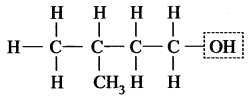This compound contains -OH group, i.e. alcohol.

Carbon And Its Compounds Class 10 In Tamil Question 3.
The secondary suffix used in IUPAC nomenclature of an aldehyde is ______.
(a) – ol
(b) – oic acid
(c) – al
(d) – one.
Answer:
(c) – al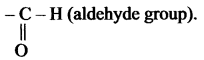Suffix ‘al’ is used to exhibit the aldehyde group.

Chapter Carbon And Its Compounds Class 10 Question 4.
Which of the following pairs can be the successive members of a homologous series?
(a) C3H8 and C4H10
(b) C2H2 and C2H4
(c) CH4 and C3H6
(d) C2H5OH and C4H8OH
Answer:
(a) C3H8 and C4H10

Class 10 Science Ch 11 Question 5.
C2H5OH + 3O2 → 2CO2 + 3H2O is a ______.
(a) Reduction of ethanol
(b) Combustion of ethanol
(c) Oxidation of ethanoic acid
(d) Oxidation of ethanol.
Answer:
(b) Combustion of ethanol
Hint: Above chemical reaction is an example of combustion reaction because it produces CO2 and H2O on the action of O2.

Characteristics Of Homologous Series Class 11 Question 6.
Rectified spirit is an aqueous solution which contains about ………… of ethanol.
(a) 95.5%
(b) 15.5%
(c) 55.5%
(d) 45.5%
Answer:
(a) 95.5%

Science Class 10 Carbon And Its Compounds Question 7.
Which of the following are used as anaesthetics?
(a) Carboxylic acids
(b) Ethers
(c) Esters
(d) Aldehydes.
Answer:
(b) Ethers

11 Carbon Chain Name Question 8.
TFM in soaps represents ………… content in soap.
(a) mineral
(b) vitamin
(c) fatty acid
(d) carbohydrate
Answer:
(c) fatty acid

Carbon And Its Compounds Pdf Question 9.
Which of the following statements is wrong about detergents?
(a) It is a sodium salt of long – chain fatty acids
(b) It is sodium salts of sulphonic acids
(c) The ionic part in a detergent is $$-\mathrm{SO}_{3}-\mathrm{Na}^{+}$$
(d) It is effective even in hard water.
Answer:
(a) It is a sodium salt of long-chain fatty acids
Hint: Detergents are not sodium salt of long – chain fatty acids. Actually soaps are sodium salts of long – chain fatty acids.

II. Fill in the blanks.

Class 10 Science Ch 11 Solutions Question 1.
An atom or a group of atoms which is responsible for chemical characteristics of an organic compound is called ______.
Answer:
Functional group.

Soap And Detergent Class 10 Notes Question 2.
The general molecular formula of alkynes is ______.
Answer:
CnH2n-2.

Class 10 Science Chapter 11 Notes Question 3.
In IUPAC name, the carbon skeleton of a compound is represented by ______ (root word / prefix / suffix)
Answer:
Root word.

Carbon And Its Compounds Class 10 Question Bank Pdf Question 4.
______ compounds decolourize bromine water. (Saturated/Unsaturated)
Answer:
Unsaturated.

Carbon And Its Compounds Class 10 Full Chapter Video Download Question 5.
Dehydration of ethanol by concentrated Sulphuric acid forms ________ (ethene/ethane)
Answer:
Ethene.

Define Catenation Class 10 Question 6.
100% pure ethanol is called ______.
Answer:
Absolute alcohol.

Carbon And Its Compounds Solutions Question 7.
Ethanoic acid turns ________ litmus to ______.
Answer:
Blue, red.

Characteristics Of Homologous Series Class 10 Question 8.
The alkaline hydrolysis of fatty acids is termed as ______.
Answer:
Saponification.

Question 9.
Biodegradable detergents are made of ______ (branched / straight) chain hydrocarbons.
Answer:
Straight.

III. Match the following

Question 1.

 i. Functional group –OH a. Benzene ii. Heterocyclic b. Potassium stearate iii. Unsaturated c. Alcohol iv. Soap d. Furan v. Carbocyclic e. Ethene

Answer:
i – c, ii – d, iii – e, iv – b , iv – a.
Hint:
i. Alcohol (-OH) is a functional group.
ii. Furan is a heterocyclic compound because it contains an oxygen atom in the cyclic ring.
iii. Ethene contains a double bond, so it is unsaturated.
iv. Soap is potassium/sodium stearate.
v. Benzene is a six-membered carbon atom ring, so it is carbocyclic.

IV. Assertion and Reason.

Answer the following questions using the data given below:
(i) A and R are correct, R explains the A.
(ii) A is correct, R is wrong.
(iii) A is wrong, R is correct.
(iv) A and R are correct, R doesn’t explain A.

Question 1.
Assertion: Detergents are more effective cleansing agents than soaps in hard water.
Reason: Calcium and magnesium salts of detergents are water – soluble.
Answer:
(ii) A is correct, R is wrong.

Question 2.
Assertion: Alkanes are saturated hydrocarbons.
Reason: Hydrocarbons consist of covalent bonds.
Answer:
(iv) A and R are correct, R doesn’t explain A.
Alkanes are saturated hydrocarbons due to the presence of single bonds.

V. Short Answer Questions.

Question 1.
Name the simplest ketone and give its structural formula.
Answer:
Simplest ketone contains three carbon atom chain with the molecular formula C3H6O.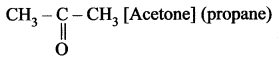Question 2.
Classify the following compounds based on the pattern of the carbon chain and give their structural formula:

1. Propane
2. Benzene
3. Cyclobutane
4. Furan.

Answer:

1. Propane is an open chain or a cyclic compound because it contains an open chain.
CH3-CH3-CH3 [Propane].
2. Benzene is a carbocyclic compound because it contains carbon atoms cyclic ring of 6 atoms.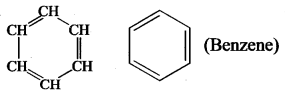3. Cyclobutane is a carbocyclic compound.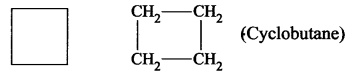4. Furan is a heterocyclic compound because in the cyclic chain one atom is oxygen atom. HC – CH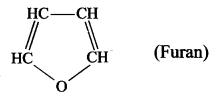Question 3.
How is ethanoic acid prepared from ethanol? Give the chemical equation.
Answer:
Ethanoic acid can be prepared by oxidation of ethanol in the presence of alkaline potassium permanganate of acidified potassium dichromate.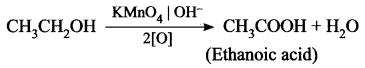Question 4.
How do detergents cause water pollution? Suggest remedial measures to prevent this pollution?
Answer:
(i) Some detergents having a branched hydrocarbon chain are not fully biodegradable by microorganism present in water and they cause water pollution.
(ii) Remedial Action: We have to use biodegradable detergents which have linear hydrocarbon chains.

Question 5.
Differentiate soaps and detergents.
Answer:

 Soaps Detergents 1. It is a sodium salt of long – chain fatty acids. 1. It is a sodium salt of sulphonic acids. 2. Its effectiveness is reduced when used in hard water. 2. It is effective even in hard water. 3. Soaps are biodegradable. 3. Most of the detergents are non-biodegradable.

VI. Long Answer Questions.

Question 1.
What is called a homologous series? Give any three of its characteristics?
Answer:
A homologous series is a group or a class of organic compounds having same general formula and similar chemical properties in which the successive members differ by a -CH2 group.
Characteristics of homologous series:

1. Each member of the series differs from the preceeding or succeeding member by one methylene group (-CH2) and hence by a molecular mass of 14 amu.
2. All members of a homologous series contain the same elements and functional group.
3. They are represented by a general molecular formula. Eg: Alkanes, C2H2n+2.
4. The members in each homologous series show a regular gradation in their physical properties with respect to their increase in molecular mass.
5. Chemical properties of the members of a homologous series are similar.
6. All the members can be prepared by a common method.

Question 2.
Arrive at, systematically, the IUPAC name of the compound: CH3 – CH2 – CH2 – OH.
Answer:

• Step 1: The parent chain consists of 4 carbon atoms. The root word is ‘but’.
• Step 2: All are single bonds between the carbon atoms of the chain. So the primary suffix is ‘ane’.
• Step 3: Since the compound contains the -OH group, it is an alcohol. The secondary suffix is ‘ol’.
But + ane + ol = Butan-ol = Butanol.

Question 3.
How is ethanol manufactured from sugarcane?
Answer:
Fermentation method can be used to obtain ethanol from sugarcane. Actually, in industries, C2H5OH is prepared by the fermentation of molasses, which is a by – product obtained during the manufacture of sugar from sugarcane.
It is converted into ethanol by following steps:

• Dilution of molasses
• Addition of nitrogen source
• Addition of yeast
• Distillation of wash.

Chemical Reductions: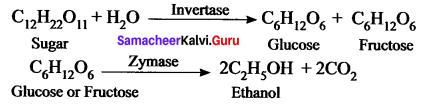Question 4.
Give the balanced chemical equation of the follows ing reactions:
(i) Neutralization of NaOH with ethanoic acid.
(ii) Evolution of carbon dioxide by the action of ethanoic acid with NaHCO3
(iii) Oxidation of ethanol by acidified potassium dichromate.
(iv) Combustion of ethanol.
Answer: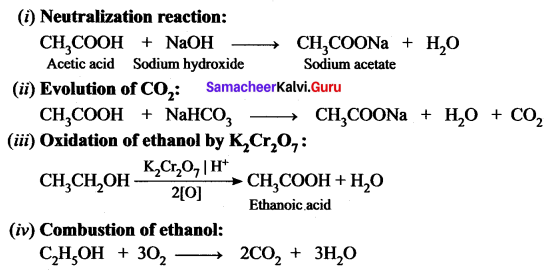Question 5.
Explain the mechanism of cleansing action of soap.
Answer:
(i) A soap molecule contains two chemically distinct parts that interact differently with water. It has one polar end, which is a short head with a carboxylate group (-COONa) and one non-polar end having the long tail made of the hydrocarbon chain.

(ii) The polar end is hydrophilic (Water loving) in nature and this end is attracted towards water. The non-polar end is hydrophobic (Water hating) in nature and it is attracted towards dirt or oil on the cloth, but not attracted towards water. Thus, the hydrophobic part of the soap molecule traps the dirt and the hydrophilic part makes the entire molecule soluble in water.

(iii) When a soap or detergent is dissolved in water, the molecules join together as clusters called ‘micelles’. Their long hydrocarbon chains attach themselves to the oil and dirt. The dirt is thus surrounded by the non-polar end of the soap molecules. The charged carboxylate end of the soap molecules makes the micelles soluble in water. Thus, the dirt is washed away with the soap.

VII. HOT Questions.

Question 1.
The molecular formula of an alcohol is C4H10O. The locant number of its -OH group is 2.
(i) Draw its structural formula.
(ii) Give its IUPAC name.
(iii) Is it saturated or unsaturated?
Answer: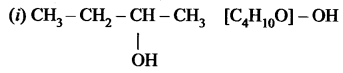the group is placed in the secondary carbon atom.

(ii) Butan-2-ol

(iii) In butan-2-al all bonds are single bonds. So, this is a saturated compound.

Question 2.
An organic compound ‘A’ is widely used as a preservative and has the molecular formula C2H4O2. This compound reacts with ethanol to form a sweet – smelling compound ‘B’.

1. Identify the compound ‘A’.
2. Write the chemical equation for its reaction with ethanol to form compound ‘B’.
3. Name the process.

Answer:

1. Acetic acid (CH3COOH) is widely used as a preservative.
2. CH3COOH reacts with ethanol to form fruity smell ester, this reaction is known as an esterification reaction.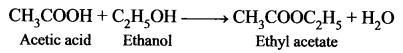3. Esterification process.

### Samacheer Kalvi 10th Science Carbon and its Compounds Additional Questions

I. Choose the best answer.

Question 1.
Most of the organic compounds are insoluble in ______.
(a) Ether
(b) CCl4
(c) Toluene
(d) Water.
Answer:
(d) Water.

Question 2.
Which is/are unsaturated compounds among the following?
(a) Methane
(b) Ethene
(c) Propyne
(d) Both (b) and (c)
Answer:
(d) Both (b) and (c)

Question 4.
Pick out the saturated compound form the following ______.
(a) propane
(b) propene
(c) propyne
(d) butene.
Answer:
(a) propane

Question 4.
The alkane with six carbon atoms is known as:
(a) Propane
(b) Pentane
(c) Hexene
(d) Hexane
Answer:
(d) Hexane

Question 5.
Which one of the following is an example for the carbocyclic compound?
(a) Benzene
(b) Toluene
(c) Propane
(d) Furan.
Answer:
(d) Furan.

Question 6.
Structure of cyclobutane is ______.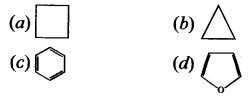Answer: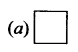Question 7.
The general formula for ketones is written as:
(a) R – OH
(b) R-COOR
(c) ROR
(d) RCOR
Answer:
(d) RCOR

Question 8.
General formula for alkane is ______.
(a) CnH2n
(b) CnH2n+2
(c) CnH2n-2
(d) CnHn
Answer:
(b) CnH2n+2

Question 9.
Which one of the following is a general formula for an alkene?
(a) CnH2n
(b) CnH2n+2
(c) CnH2n-2
(d) CnHn
Answer:
(a) CnH2n

Question 10.
Hand sanitizers contain:
(a) Ethanol
(b) Ethanal
(c) Ethanoic acid
(d) Ethane
Answer:
(a) Ethanol

Question 11.
Lower hydrocarbons are ______ state at room temperature.
(a) solid
(b) liquid
(c) gaseous
(d) viscous.
Answer:
(c) gaseous

Question 12.
Reagent which distinguishes alkane and alkene is ______.
(a) Br2 / H2O
(b) H2O
(c) C2H5OH
(d) Ether.
Answer:
(a) Br2 / H2O

Question 13.
Pick out the functional group for alcohol ______.
(a) -CHO
(b) -COOH
(c) -OH
(d) -OR.
Answer:
(c) -OH

Question 14.
-COOH is a functional group of ______.
(a) carboxylic acid
(b) ester
(c) ether
(d) aldehyde.
Answer:
(a) carboxylic acid

Question 15.
Which one of the following is the correct sequence to get the IUPAC name of the cojnpound?
(a) Prefix + Root word + Suffix → IUPAC name
(b) Prefix + Suffix + Root word → IUPAC name
(c) Suffix + Root word + Prefix → IUPAC name
(d) Root word + Prefix + Suffix → IUPAC name.
Answer:
(a) Prefix + Root word + Suffix → IUPAC name

Question 16.
What is the root word, if the compound has nine number of carbon atoms?
(a) Meth-
(b) Oct-
(c) Non-
(d) Dec-.
Answer:
(c) Non-

Question 17.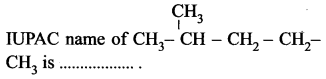(a) pentane
(b) 2-methyl pentane
(c) 4-methyl pentane
(d) 1, 1-dimethyl butane.
Answer:
(b) 2-methyl pentane

Question 18.
Dehydration of ethanol gives ______.
(a) Ethane
(b) Ethene
(c) Ethyne
(d) no reaction.
Answer:
(b) Ethene

Question 19.
Dehydration of ethanol gives ______.
(a) Ethanol
(b) Ethanoic acid
(c) Ethyne
(d) Ethene.
Answer:
(a) Ethanol

Question 20.
Power alcohol is a mixture of ______.
(a) Ethanol + Methane
(b) Ethanol + water
(c) Ethanol + Petrol
(d) Ethanol + Pyridine.
Answer:
(c) Ethanol + Petrol

Question 21.
Which one of the following has sour in taste?
(a) Ethanol
(b) Ethanoic acid
(c) Ethanal
(d) Ethyne.
Answer:
(b) Ethanoic acid

Question 22.
Decarboxylation of ethanoic acid is ______.
(a) Ethane
(b) Methane
(c) Propane
(d) Ethanol.
Answer:
(b) Methane

Question 23.
______ compounds hold the key to plant and animal life on the earth.
(a) Sulphur
(b) Carbon
(c) Nitrogen
(d) Boron.
Answer:
(b) Carbon

Question 24.
All living organisms are made of ______ atoms.
(a) phosphorous
(b) sodium
(c) carbon
(d) sulphur.
Answer:
(c) carbon

Question 25.
Which of the following is not the characteristic of carbon?
(a) carbon form allotropes
(b) carbon is a tetravalent atom
(c) carbon is a metal
(d) catenation is possible in carbon.
Answer:
(c) carbon is a metal

Question 26.
Which one is the characteristic of carbon compounds?
(a) Carbon compounds have high melting and boiling point.
(b) Carbon compounds show isomerism.
(c) Carbon compounds are electrovalent compounds.
(d) Carboncompoundsarenotcombustible.
Answer:
(b) Carbon compounds show isomerism.

Question 27.
The hydrocarbons containing at least one carbon to carbon double bond are called ______.
(a) paraffin
(b) alkyne
(c) alkene
(d) alkane.
Answer:
(c) alkene

Question 28.
Decolourisation of bromine takes place in ______.
(a) CH2 = CH2
(b) CH4
(c) CH3 – CH3
(d) CH3 – CH2OH.
Answer:
(a) CH2 = CH2

Question 29.
The hydrocarbons containing carbon to carbon triple bond are called ______.
(a) Alkane
(b) Alkyne
(c) Paraffin
(d) Alkene.
Answer:
(b) Alkyne

Question 30.
The IUPAC name of $$\mathrm{H}_{3} \mathrm{C}-\mathrm{CH}_{2}-\mathrm{C} \equiv \mathrm{CH}$$ is ______.
(a) 2-butyne
(b) But-2-ene
(c) 1-butyne
(d) But-1-ene.
Answer:
(c) 1-butyne

Question 31.
The common name of methanoic acid is ______.
(a) Acetic acid
(b) Formic acid
(c) Propionic acid
(d) Butyric acid.
Answer:
(b) Formic acid

Question 32.
Molasses contains ______.
(a) 50 % glucose
(b) 90 % sucrose
(c) 30 % sucrose
(d) 50 % fructose.
Answer:
(c) 30 % sucrose

Question 33.
______ acts as food for yeast during the fermentation of molasses?
(a) Ammonium sulphate
(b) Dilute H2SO4
(c) Ammonium nitrate
(d) Quick lime.
Answer:
(a) Ammonium sulphate

Question 34.
The rectified spirit contains ______.
(a) 100 % ethanol
(b) 50 % ethanol + 50 % water
(c) 95.5 % ethanol and 4.5 % water
(d) Ethanol + pyridine.
Answer:
(c) 95.5 % ethanol and 4.5 % water

Question 35.
The enzyme used in the conversion of glucose into ethanol is ______.
(a) invertase
(b) maltase
(c) diastase
(d) zymase.
Answer:
(d) zymase.

Question 36.
100% pure ethanol is known as ______.
(a) power alcohol
(b) rectified spirit
(c) absolute alcohol
(d) denatured spirit.
Answer:
(c) absolute alcohol

Question 37.
Denatured spirit is obtained by mixing ethanol with ______.
(a) pyridine
(b) petrol
(c) methanol
(d) quicklime.
Answer:
(a) pyridine

Question 38.
The reaction has taken place when ethanol is heated with Conc. H2SO4 of 443K is ______.
(a) oxidation
(b) reduction
(c) intermolecular dehydration
(d) intramolecular dehydration.
Answer:
(d) intramolecular dehydration.

Question 39.
The reaction took place when ethanol is heated with Conc. H2SO4 at 413K is ______.
(a) intermolecular dehydration
(b) hydrogenation
(c) oxidation
(d) intramolecular dehydration.
Answer:
(a) intermolecular dehydration

Question 40.
Which reagent is used to identify alcohol consumed persons?
(a) CH3COOH
(b) CaO
(c) K2Cr2O7
(d) H2SO4
Answer:
(c) K2Cr2O7

Question 41.
The reaction of ethanol with ethanoic acid in the presence of Conc. H2SO4 is known as ______.
(a) etherification
(b) esterification
(c) dehydrogenation
(d) dehydration.
Answer:
(b) esterification

Question 42.
Which reagent is used to convert ethanol to acetaldehyde?
(a) Conc.H2SO4
(b) Acidified
(c) Alkaline KMnO4
(d) Copper.
Answer:
(d) Copper.

Question 43.
Which compound is used as an anti-freeze in automobile radiators?
(a) Acetic acid
(b) Ethyl ethanoate
(c) Ethanol
(d) Acetaldehyde.
Answer:
(c) Ethanol

Question 44.
The organic compound used in cough syrups and in digestive syrups is ______.
(a) ethanoic acid
(b) ethyl ethanoate
(c) methanol
(d) ethanol.
Answer:
(d) ethanol.

Question 45.
The organic compound that depresses the central nervous system after consumption is ______.
(a) ethanol
(b) methanol
(c) acetic acid
(d) ethyl ethanoate.
Answer:
(b) methanol

Question 46.
The organic compound used for coagulating rubber from latex is ______.
(a) methanoic acid
(b) ethanoic acid
(c) ethanol
(d) methanol.
Answer:
(b) ethanoic acid

Question 47.
The alcohol that is poisonous in nature is ______.
(a) methanol
(b) ethanol
(c) benzyl alcohol
(d) phenol.
Answer:
(a) methanol

Question 48.
In a homologous series, the successive compounds differ by a ______ group.
(a) CH2
(b) CH
(c) CH3
(d) C2H5
Answer:
(a) CH2

Question 49.
The fermented liquid wash contains ____ % alcohol.
(a) 90
(b) 8 – 25
(c) 15 – 18
(d) 40 – 60.
Answer:
(c) 15 – 18

Question 50.
Carbon has the ability to form ______ bonds.
(a) ionic
(b) covalent
(c) electrovalent
(d) dative.
Answer:
(b) covalent

Question 51.
Decarboxylation is removal of ______.
(a) CO
(b) CO2
(c) H2
(d) $$\mathrm{CO}_{3}^{-}$$.
Answer:
(b) CO2

Question 52.
The enzyme used in the conversion of molasses into glucose and fructose is ______.
(a) zymase
(b) diastase
(c) invertase
(d) maltase.
Answer:
(c) invertase

II. Fill in the blanks.

Question 1.
Organic compounds are _____ reactive.
Answer:
Less.

Question 2.
The reaction involving organic compounds proceed at ______ rates.
Answer:
Slower.

Question 3.
Organic compounds form ____ bonds in nature.
Answer:
Covalent.

Question 4.
If organic compounds contain carbon and other atoms like O, N, S etc., these compounds are called ______.
Answer:
Heterocyclic compounds.

Question 5.
The organic compounds that are composed of only carbon and hydrogen atoms are called ______.
Answer:
Hydrocarbons.

Question 6.
Alkynes are the most reactive due to the presence of the ______.
Answer:
Triple bond.

Question 7.
A series of compounds containing the same functional group is called ______.
Answer:
Class of organic compounds.

Question 8.
______ is used as an anti-freeze in automobile radiators.
Answer:
Ethanol.

Question 9.
Vanilla beans solution is made up of ______ and ______.
Answer:
Ethanol, water.

Question 10.
Soda – lime is a mixture of ______.
Answer:
3 parts of NaOH(s) + 1 part of CaO(s).

Question 11.
_____ is used as a flavouring agent and preservative.
Answer:
Ethanoic acid.

Question 12.
Formaldehyde is used as a ______.
Answer:
Disinfectant.

Question 13.
______ is a Anaesthetic agent.
Answer:
Ethers.

Question 14.
All the cooking oils and lipids contain ______.
Answer:
Esters.

Question 15.
The most commonly used alkali for preparation of soap is ______.
Answer:
Sodium hydroxide.

Question 16.
______ process helps to manufacture of soap.
Answer:
Kettle.

Question 17.
________ are salts of sulphonic acids.
Answer:
Detergents.

Question 18.
When soap or detergent is dissolved in water, the molecules join together as clusters called ______.
Answer:
Micelles.

Question 19.
A soap which has ______ TFM is a good bathing soap.
Answer:
Higher.

Question 20.
______ is present in many fruits.
Answer:
Acetic acid.

Question 21.
______ are sodium salts of long-chain carboxylic acids.
Answer:
Soap.

Question 22.
Unsaturated carbon compounds undergo _____ reactions whereas saturated carbon compounds undergo ______ reactions.
Answer:
Addition, substitution.

Question 23.
Each member of the homologous series differ from the succeeding member by a common difference of _____ and by a molecular mass of ______.
Answer:
CH2, 14 amu.

Question 24.
Saturated hydrocarbons were earlier named as ______ and by IUPAC system they are named as ______.
Answer:
Paraffin, Alkanes.

Question 25.
Alkenes have the general formula ______ and they were previously called ______.
Answer:
CnH2n, olefins.

Question 26.
The slow chemical change that takes place in complex organic compounds by the action of _______ leading to the formation of simple molecules is called ______.
Answer:
Enzymes, fermentation.

Question 27.
A mixture contains 95.5% ethanol and 4.5% water is called ______ and 100% pure ethanol is called ______.
Answer:
Rectified spirit, absolute alcohol.

Question 28.
Ethanol when heated with Conc. H2SO4 at 443K gives ______ and at 413K gives ______ as products.
Answer:
Ethene, diethyl ether.

Question 29.
During the oxidation of ethanol with acidified K2Cr2O7, the _____ colour changes to ______ colour.
Answer:
Orange, green.

Question 30.
The compound formed by the reaction of an alcohol with a carboxylic acid is known as ______ and the reaction is called ______.
Answer:
Ester, esterification.

Question 31.
______ is used as a preservative for biological specimens and ______ is used as a preventative in food and fruit juices.
Answer:
Ethanol, Ethanoic acid.

III. Match the following.

Question 1.

 1. CnH2n+2 (a) Alkene 2. CnH2n (b) Alcohol 3. CnH2n-2 (c) Alkane 4. ROH (d) Alkyne

Answer:
1. (c), 2. (a), 3. (d), 4. (b).

Question 2.

 1. CH3 – CH2OH (a) Ethanal 2. CH3 – COOH (b) Ethanol 3. CH3 – CHO (c) Propanone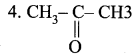(d) Ethanoic acid

Answer:
1. (b), 2. (d), 3. (a), 4. (c).

Question 3.

 1. Methylated spirit (a) Ethanol + water 2. Power alcohol (b) Ethanol + pyridine 3. Denatured spirit (c) Ethanol + petrol 4. Rectified spirit (d) Ethanol + methanol

Answer:
1. (d), 2. (c), 3. (b), 4. (a).

Question 4.

 Compound Common Name IUPAC Name CH2 = CH2 Acetylene Butane $$\mathrm{CH}_{3}-\mathrm{C} \equiv \mathrm{C}-\mathrm{CH}_{3}$$ n-butane Ethene CH3 – CH2 – CH2 – CH3 Dimethyl acetylene Ethyne $$\mathrm{CH} \equiv \mathrm{CH}$$ Ethylene 2 – butyne

Answer:

 Compound Common Name IUPAC Name CH2 = CH2 Ethylene Ethene $$\mathrm{CH}_{3}-\mathrm{C} \equiv \mathrm{C}-\mathrm{CH}_{3}$$ Dimethyl acetylene 2 – butyne CH3 – CH2 – CH2 – CH3 n-butane Butane $$\mathrm{CH} \equiv \mathrm{CH}$$ Acetylene Ethyne

Question 5.

 1. Alcohol (a) >C = O 2. Aldehyde (b) -COOH 3. Ketone (c) -CHO 4. Carboxylic acid (d) -HO

Answer:
1. (d), 2. (c), 3. (a), 4. (b).

Question 6.

 1. Ethene (a) C3H4 2. Ethane (b) C3H6 3. Propyne (c) C2H4 4. Propene (d) C2H6

Answer:
1. (c), 2. (d), 3. (a), 4. (b).

Question 7.

 1. CH3OH (a) Dimethyl Ketone 2. CH3CHO (b) Acetic acid 3. CH3COCH3 (c) Acetaldehyde 4. CH3COOH (d) Methyl alcohol

Answer:
1. (d), 2. (c), 3. (a), 4. (b).

Question 8.

 Common Name Formula 1. Methane (a) CH3CH2CH2CH3 2. Ethane (b) CH3CH2CH3 3. Propane (c) CH4 4. n-Butane (d) CH3CH3

Answer:
1. (c), 2 .(d), 3. (b), 4. (a).

Question 9.

 1. Acyclic compounds (a) Cyclobutene 2. Alicyclic compounds (b) Ethene 3. Homocyclic aromatic compounds (c) Pyridine 4. Heterocyclic compounds (d) Benzene

Answer:
1. (b), 2. (a), 3. (d), 4. (c).

Question 10.

 1. Sodium silicate (a) glow to the clothes 2. Fluorescent whitening agent (b) removal of certain stains 3. Sodium perborate (c) prevent the caking of the detergent powder 4. Sodium sulphate (d) prevents corrosion

Answer:
1. (d), 2. (a), 3. (b), 4. (c).

IV. State True or False. If false give the correct statement.

Question 1.
Carbon circulates through air, plants, animals and soil by means of complex reactions is called the Kreb cycle.
Answer:
False.
Correct statement: Carbon circulates through air, plants, animals and soil by means of complex reactions is called the carbon cycle.

Question 2.
Carbon atoms form the building blocks of living organisms.
Answer:
True.

Question 3.
The characteristics of a carbon atom by virtue of which it forms four covalent bonds are referred to as catenation.
Answer:
False.
Correct statement: The characteristic of a carbon atom by virtue of which it forms four covalent bonds is referred to as tetravalency of carbon.

Question 4.
Carbon compounds show isomerism and possess the characteristic property catenation.
Answer:
True.

Question 5.
Carbon compounds have high melting and boiling points because of their electrovalent nature.
Answer:
False.
Correct statement: Carbon compounds have low melting and boiling points because of their covalent nature.

Question 6.
Alcohols react with sodium metal to liberate oxygen gas.
Answer:
False.
Correct statement: Alcohols react with sodium metal to liberate hydrogen gas.

Question 7.
All members of each homologous series contain different elements and different functional groups.
Answer:
False.
Correct statement: All members of each homologous series contain the same elements and same functional groups.

Question 8.
The chemical properties of the members of each homologous series are similar.
Answer:
True.

Question 9.
Decolourisation of bromine takes place in saturated compounds.
Answer:
False.
Correct statement: Decolourisation of bromine takes place in unsaturated compounds.

Question 10.
Molasses is a dark coloured syrupy liquid left after the crystallization of sugar from sugarcane juice.
Answer:
True.

Question 11.
Rectified spirit on heating with Cone. H2SO4 for about 5 to 6 hours and allowed to stand for 12 hours to get absolute alcohol.
Answer:
False.
Correct statement: Rectified spirit on heating with quicklime for about 5 to 6 hours and allowed to stand for 12 hours to get absolute alcohol.

Question 12.
Ethanol is a clear liquid with burning taste whereas ethanoic acid is a colourless liquid with a sour taste.
Answer:
True.

Question 13.
Ethanol affects the optic nerve causing blindness.
Answer:
False.
Correct statement: Methanol affects the optic nerve causing blindness.

Question 14.
Consumption of ethanol slows down the metabolism of our body and depresses the central nervous system.
Answer:
True.

Question 15.
On cooling, pure ethanol is frozen to form ice – like flakes.
Answer:
False.
Correct statement: On cooling, pure ethanoic acid is frozen to form ice – like flakes.

Question 16.
Methanol is oxidised to methanal in the liver and reacts with components of cells.
Answer:
True.

Question 17.
Removal of carbon dioxide is known as dehydrogenation.
Answer:
False.
Correct statement: Removal of carbon dioxide is known as decarboxylation.

Question 18.
Organic compounds have a high molecular weight and a simple structure.
Answer:
False.
Correct statement: Organic compounds have a high molecular weight and a complex structure.

Question 19.
The organic compound is less reactive than inorganic compounds.
Answer:
True.

Question 20.
Answer:
Organic compounds have high melting and boiling points.
Answer:
False.
Correct statement: Organic compounds have low melting and boiling points.

Question 21.
Furan is a carbocyclic compound.
Answer:
False.
Correct statement: Furan is a heterocyclic compound.

Question 22.
The boiling point of hydrocarbons increases with an increase in the number of carbon atoms.
Answer:
True.

Question 23.
Saturated compounds, decolourise the bromine water.
Answer:
False.
Correct statement:

• Unsaturated compounds, decolourise the bromine water.
• Saturated compounds do not decolourise bromine water.

Question 24.
Chemical properties of the members of a homologous series are similar.
Answer:
True.

Question 25.
IUPAC stands for International Union of Pure and Analytical Chemistry.
Answer:
False.
Correct statement: IUPAC stands for International Union of Pure and Applied Chemistry.

Question 26.
Hard soaps are used for cleaning the body.
False.
Correct statement: Soft soaps are used for cleaning the body.

Question 27.
Hard water limits the cleaning action of soap.
Answer:
True.

Question 28.
Soap forms a scum in hard water.
Answer:
True.

Question 29.
Soap has greater foaming capacity.
Answer:
False.
Correct statement: Soap has a poor foaming capacity.

Question 30.
Most of the detergents are biodegradable.
Answer:
False.
Correct statement: Most of the detergents are non-biodegradable.

V. Assertion and Reason.

Question 1.
Assertion (A): Carbon compounds hold the key to plant and animal life on the earth.
Reason (R): Carbon circulates through air, plants, animals and soil by means of complex reactions.
(a) Both (A) and (R) are correct
(b) Both (A) and (R) are wrong
(c) (A) is correct but (R) is wrong
(d) (A) is wrong but (R) is correct.
Answer:
(a) Both (A) and (R) are correct

Question 2.
Assertion (A): Carbon chemistry is called as living chemistry.
Reason (R): The carbon atoms form the building blocks of living organisms and carbon combined with other atoms decide life on earth.
(a) Both (A) and (R) are wrong
(b) Both (A) and (R) are correct
(c) (A) is correct but (R) is wrong
(d) (A) is wrong but (R) is correct.
Answer:
(b) Both (A) and (R) are correct

Question 3.
Assertion(A): C4+ cation formation is easy.
Reason (R): Carbon can lose four electrons to form C4+ cation require less amount of energy.
(a) Both (A) and (R) are correct
(b) (A) is wrong but (R) is correct
(c) (A) is correct but (R) is wrong
(d) Both (A) and (R) are wrong.
Answer:
(d) Both (A) and (R) are wrong.

Question 4.
Assertion (A): Methane is formed when four electrons of carbon are shared with four hydrogen atoms.
Reason (R): This characteristic of a carbon atom by virtue of which it forms four covalent bonds is referred to as tetra valency of carbon.
(a) (A) is correct and (R) explains (A)
(b) Both (A) and (R) are wrong
(c) (A) is correct but (R) does not explain (A)
(d) (A) is wrong but (R) is correct.
Answer:
(a) (A) is correct and (R) explains (A)

Question 5.
Assertion (A): Diamond is a rigid substance and it is very hard.
Reason (R): In diamond, each carbon atom is bonded to three other carbon atoms in the same place giving hexagonal layers held together by weak van der Waals forces.
(a) Both (A) and (R) are correct
(b) (A) is correct but (R) does not explain (A)
(c) Both (A) and (R) are wrong
(d) (A) is wrong but (R) is correct.
Answer:
(b) (A) is correct but (R) does not explain (A)

Question 6.
Assertion (A): Graphite is a good conductor of electricity, unlike other non – metals.
Reason (R): Graphite has free electrons in it.
(a) Both (A) and (R) are correct
(b) (A) is correct but (R) does not explain (A)
(c) (A) is wrong but (R) is correct
(d) Both (A) and (R) are wrong.
Answer:
(a) Both (A) and (R) are correct

Question 7.
Assertion (A): Carbon combines with many other elements to form various stable compounds.
Reason (R): The stability of carbon compounds is due to the small size of carbon which enables the nucleus to hold on to the shared pair of electrons strongly.
(a) Both (A) and (R) are wrong
(b) Both (A) and (R) are correct
(c) (A) is correct but (R) is wrong
(d) (A) is wrong but (R) is correct.
Answer:
(b) Both (A) and (R) are correct.

Question 8.
Assertion (A): Carbon compounds have low melting and boiling points and they are easily combustible.
Reason (R): Carbon compounds are electrovalent compounds.
(a) Both (A) and (R) are correct
(b) (A) is correct but (R) is wrong
(c) Both (A) and (R) are wrong
(d) (A) is wrong but (R) is correct.
Answer:
(b) (A) is correct but (R) is wrong

Question 9.
Assertion (A): Ethanol has a much higher boiling point than ethane.
Reason (R): Presence of hydrogen bond in a molecule increases the boiling point.
(a) Both (A) and (R) are correct, (R) explains (A)
(b) (A) is correct but (R) is wrong
(c) (A) is wrong but (R) is correct
(d) Both (A) and (R) are correct, but (R) doesn’t explain (A).
Answer:
(a) Both (A) and (R) are correct, (R) explains (A)

Question 10.
Assertion (A): Hard water limits the cleaning action of soap.
Reason (R): When combined with soap, hard water develops a thin layer.
(a) Both (A) and (R) are correct, (R) explains (A)
(b) (A) is correct but (R) is wrong
(c) (A) is wrong but (R) is correct
(d) Both (A) and (R) are correct, but (R) doesn’t explain (A).
Answer:
(a) Both (A) and (R) are correct, (R) explains (A)

VI. Short Answer Questions.

Question 1.
How will you test to identify saturated and unsaturated compounds?
Answer:
Test to identify saturated and unsaturated compounds:

• Take the given sample solution in a test tube.
• Add a few drops of bromine water and observe any characteristic change in colour.
• If the given compound is unsaturated, it will decolourise bromine water.
• Saturated compounds do not decolourise bromine.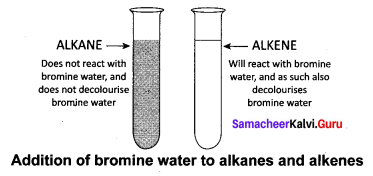Question 2.
What is a functional group?
Answer:
A functional group is an atom or group of atoms in a molecule, which gives its characteristic chemical properties.

Question 3.
What is a locant number?
Answer:
Number the carbon atoms of the parent chain, beginning at the closest end of the substituent or functional group. These are called locant numbers.

Question 4.
Obtain the IUPAC name of the following compounds systematically.
(i) CH3 – CH2 – CH2 – CH2 – CH3Answer:
(i) CH3 – CH2 – CH2 – CH2 – CH3

• Step 1: It is a five-carbon chain and hence the root word is ‘Pent’. (Rule 1)
• Step 2: All the bonds between carbon atoms are single bonds, and thus the suffix is ‘ane’. So, its name is Pent + ane = Pentane• Step 1: The longest chain contains five carbon atoms and hence the root word is ‘Pent’.
• Step 2: There is a substituent. So, the carbon chain is numbered from the left end, which is closest to the substituent. (Rule 2)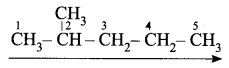• Step 3: All are single bonds between the carbon atoms and thus the suffix is ‘ane’.
• Step 4: A substituent is a methyl group and it is located at a second carbon atom. So, its locant number is 2. Thus the prefix is ‘2-Methyl’.(Rule 6).
The name of the compound is 2-Methyl + pent + ane = 2-Methylpentane

Question 5.
Mention the uses of ethanol?
Answer:

• Ethanol is used in medical wipes, as an antiseptic.
• Ethanol is used as an antifreeze in automobile radiators.
• Ethanol is used for effectively killing microorganisms like bacteria, fungi, etc., by including it in many hand sanitizers.
• Ethanol is used as an antiseptic to sterilize wounds in hospitals.
• Ethanol is used as a solvent for drugs, oils, fats, perfumes, dyes, etc.
• Ethanol is used in the preparation of methylated spirit (a mixture of 95 % of ethanol and 5 % of methanol) rectified spirit (a mixture of 95.5 % of ethanol and 4.5 % of water), power alcohol (a mixture of petrol and ethanol) and denatured spirit (ethanol mixed with pyridine).
• Ethanol is used to enhance the flavour of food extracts, for example, vanilla extract; a common food flavour, which is made by processing vanilla beans in a solution of ethanol and water.

Question 6.
What is a decarboxylation reaction?
Answer:
When a sodium salt of ethanoic acid is heated with soda lime (a solid mixture of 3 parts of NaOH and 1 part of CaO), methane gas is formed. During this reaction, the CO2 molecule is eliminated. Therefore, this reaction is called a decarboxylation reaction.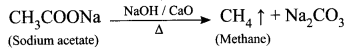Question 7.
What is soap?
Answer:
Soaps are sodium or potassium salts of some long-chain carboxylic acids, called fatty acids. Soap requires two major raw materials:

• fat
• alkali.

Question 8.
|What are the two types of soaps? Explain.
Answer:

1. Hard Soap : Soaps, which are prepared by the saponification of oils or fats with caustic soda (sodium hydroxide), are known as hard soaps. They are usually used for washing purposes.
2. Soft Soap : Soaps, which are prepared by the saponification of oils or fats with potassium salts, are known as soft soaps. They are used for cleansing the body.

Question 9.
What is soft soap?
Answer:
Soaps, which are prepared by the saponification of oils or fats with potassium salts, are known as soft soaps. They are used for cleansing the body.

Question 10.
What are the disadvantages of detergents?
Answer:

1. Some detergents having a branched hydrocarbon chain are not fully biodegradable by micro-organisms present in water. So, they cause water pollution.
2. They are relatively more expensive than soap.

Question 11.
What are biodegradable and non-biodegradable detergents?
Answer:

1. Biodegradable detergents: They have straight hydrocarbon chains, which can be easily degraded by bacteria.
2. Non – biodegradable detergents: They have highly branched hydrocarbon chains, which cannot be degraded by bacteria.

Question 12.
Mention the disadvantages of detergents.
Answer:

1. Some detergents having a branched hydrocarbon chain are not fully biodegradable by micro-organisms present in water. So, they cause water pollution.
2. They are relatively more expensive than soap.

Question 13.
What is catenation?
Answer:
Carbon has the ability to form covalent bonds with other atoms of carbon giving rise to a large number of molecules through self linking property. This property is called catenation.

Question 14.
Define isomerism. Give a suitable example.
Answer:
The phenomenon by which two or more compounds have the same molecular formula but different structural formula with the difference in properties is known as isomerism.
C2H6O. This molecular formula has two isomeric structures.

1. C2H5OH → Ethyl alcohol
2. CH3 – O – CH3 → Dimethyl ether

Question 15.
How will you differentiate saturated and unsaturated hydrocarbon compounds?
Answer:

 Saturated hydrocarbon Unsaturated hydrocarbon Saturated hydrocarbon undergoes substitution reactions in the presence of sunlight. Unsaturated hydrocarbon undergoes an addition reaction with hydrogen in the presence of nickel catalyst.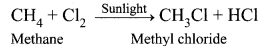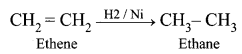Saturated hydrocarbon does not decolourise bromine water. Unsaturated hydrocarbon decolourise bromine water.

VII. Long Answer Questions

Question 1.
All living organisms are made of carbon atoms. This means that carbon atoms form the building blocks of living organisms. Justify this statement by giving physical nature of carbon and its compounds.
Answer:
Physical nature of carbon and its compounds.

1. Carbon has the ability to form covalent bonds with other atoms of carbon giving rise to a large number of molecules through self linking property which is called catenation.
2. Carbon combines with oxygen, hydrogen, nitrogen sulphur, chlorine and many other elements to form various stable compounds.
3. The stability of carbon compounds is due to the small size of carbon which enables the nucleus to hold on to the shared pair of electrons strongly.
4. Carbon compounds show isomerism, the phenomenon by which two or more compounds have the same molecular formula but different structural formula with the difference in properties.
5. Carbon compounds have low boiling points and low melting points because of their covalent nature.
6. Carbon compounds are easily combustible.

Question 2.
Explain the preparation of detergents with their ingredients.
Answer:
Detergents are prepared by adding sulphuric acid to the processed hydrocarbon obtained from petroleum. This chemical reaction result in the formation of molecules similar to the fatty acid in soap. Then, an alkali is added to the mixture to produce the ‘surfactant molecules’, which do not bond with the minerals present in the hard water, thus preventing the formation of their precipitates.

In addition to a ‘surfactant’, the modem detergent contains several other ingredients. They are listed as follows:

1. Sodium silicate, which prevents the corrosion and ensures that the detergent does not damage the washing machine.
2. Fluorescent whitening agents that give a glow to the clothes.
3. Oxygen bleaches, such as ‘sodium perborate’,enable the removal of certain stains from the cloth.
4. Sodium sulphate is added to prevent the caking of the detergent powder.
5. Enzymes are added to break down some stains caused by biological substances like blood and vegetable juice.
6. Certain chemicals that give out a pleasant smell are also added to make the clothes fragrant after they are washed with detergents.

Question 3.
Explain the classification of organic compounds.
Answer: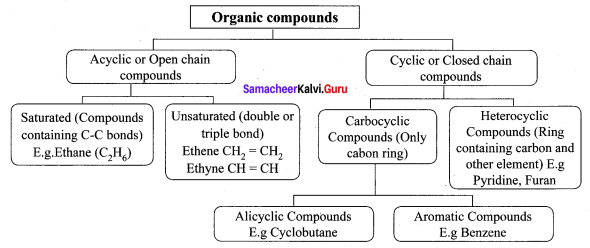Question 4.
Discuss the characteristics of hydrocarbons.
Answer:
Characteristics of hydrocarbons:

1. Lower hydrocarbons are gases at room temperature E.g. methane, ethane is gassed.
2. They are colourless and odourless.
3. The boiling point of hydrocarbons increases with an increase in the number of carbon atoms.
4. They undergo combustion reaction with oxygen to form CO2 and water.
5. Alkanes are least reactive when compared to other classes of hydrocarbons.
6. Alkynes are the most reactive due to the presence of the triple bond.
7. Alkanes are saturated whereas alkenes and alkynes are unsaturated.
8. They are insoluble in water.

Question 5.
Identify the compounds using the clues given below.
(a) This is a dark coloured syrupy liquid containing 30% of sucrose.
Answer:
Molasses.

(b) During manufacture of ethanoic acid this acts as an oxidising agent.
Answer:
Alkaline KMnO4 or acidified K2Cr2O7.

(c) This enzyme converts sucrose into glucose and fructose.
Answer:
Invertase

(d) Ethanol on dehydrogenation with Cu/573K gives this compound.
Answer:
Ethanal

(e) This compound is used as an antiseptic.
Answer:
Ethanol

VIII. HOT Questions

Question 1.
An organic compound (A) of molecular formula C2H6O reacts with sodium metal and liberates H2 gas. (A) on reaction with alkaline KMnO4 gives (B) of formula C2H6O2. The sodium salt of (B) on reaction with soda lime gives (C) a first member of alkane homologous series. Identify A, B, and C.
Answer:
(i) (A) is Ethanol: CH3 – CH2OH.
Ethanol reacts with sodium metal and liberates H2 gas.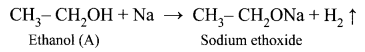(ii) Ethanol on reaction with alkaline KMnO4, oxidation takes place and the product formed is Ethanoic acid, CH3COOH. It is (B).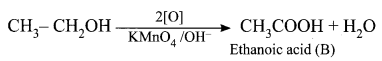(iii) The sodium salt of ethanoic acid on reaction with soda lime (NaOH + CaO) undergo decarboxylation reaction to give methane CH4 (C) the first member of alkane homologous series.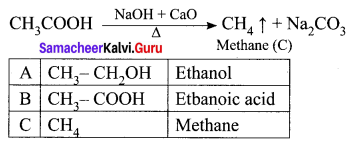Question 2.
In what way yeast is important in the fermentation process?
Answer:
Yeasts are single-celled microorganisms, belonging to the class of fungi. The enzymes present in yeasts catalyse many complex organic reactions. Fermentation is the conversion of complex organic molecules into simpler molecules by the action of enzymes. E.g. Curdling of milk.

Question 3.
Write and explain the reaction that can be used for the identification of alcohols.
Answer:
Oxidation reaction: Ethanol is oxidized to ethanoic acid with alkaline KMnO4 or acidified K2Cr2O7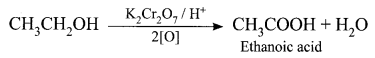During this reaction, the orange colour of K2Cr2O7 changes to green. Therefore, this reaction can be used for the identification of alcohols.

Question 4.
How dirt is washed away with the soap?
Answer:
When soap or detergent is dissolved in water, the molecules join together as clusters called micelles. Their long hydrocarbon chain attaches themselves to the oil and dirt. The dirt is thus surrounded by the non-polar end of the soap molecules. The charged carboxylate end to the soap molecules makes the micelles soluble in water. Thus the dirt is washed away with the soap.

Question 5.
Why ordinary soap is not suitable for use with hard water?
Answer:
Ordinary soaps when treated with hard water, precipitate as salts of calcium and magnesium. They appear at the surface of the cloth as sticky grey scum. Thus, the soaps cannot be used conveniently in hard water.

Test Yourself

Question 1.
Obtain the IUPAC name of the following compounds systematically.
(i) CH3CHO
(ii) CH3CH2COCH3
(iii) ClCH2 – CH2 – CH2 – CH3
Answer:
(i) CH3CHO

• Step 1: The parent chain consists of 2 carbon atoms. The root word is “Eth”.
• Step 2: All are single bonds between the carbon atom of the chain. So the primary suffix is “ane”.
• Step 3: Since the compound contains the – CHO group, it is an aldehyde. The secondary suffix is “al”.
The name of the compound is Eth + ane + al = Ethanal

(ii) CH3CH2COCH3

• Step 1: The parent chain consists of 4 carbon atoms. The root word is “But”.
• Step 2: All are single bonds between the carbon atom of the chain. So the primary suffix is “ane”.
• Step 3: Since the compound contains the – CO – group, it is a ketone group. The secondary suffix is “one”.
The name of the compound is But + ane + one = Butanone

(iii) ClCH2 – CH2 – CH2 – CH3

• Step 1: The parent chain consists of 4 carbon atoms. The root word is “But”.
• Step 2: All are single bonds between the carbon atom of the chain. So the suffix is “ane”.
• Step 3: Since the compound contains the – Cl substituent. The prefix is Chloro.
• Step 4: The locant number of – Cl is 1 and thus prefix is 1-Chloro.
The name of the compound is 1-Chloro + Butane = 1-Chlorobutane.

Hope you liked the article on Tamilnadu State Board Solutions of Class 10 Science Solutions Chapter 11 Carbon and its Compounds Questions and Answer and share it among your friends to make them aware. Keep in touch to avail latest information regarding different state board solutions instantly.